Home  │ Audio Home Page

Updated July 21, 2010.  See Document History at end for details.
Spice verfied.

## Simple DC Servos

#### Introduction

The hope of direct coupling some desireable audio circuits may be challenged by high DC offset, perhaps due to compromises made to gain some other desireable outcome.  Adding or increasing DC feedback is necessary here.  Second order servos are often used because a second passive pole is desired to decouple the typical op-amp integrator from the signal path.  Second order servos, however, require carefull juggling of gain and phase shift factors to prevent subsonic oscillation.  A simple integrator stage would do, but most would find the connection of the op-amp output into the signal path disagreeable.  Here I suggest adding to the integrator a zero matching and cancelling the pole of the second stage to create a two stage first order servo.  With a constant servo phase shift of -90°, design can proceed without worry of oscillation at all.

 Figure 1:  Bode plot shows how response of integrator with zero (red plot) adds to that of the second stage lowpass pole (green plot) to create a first order integrator response (connecting black plot).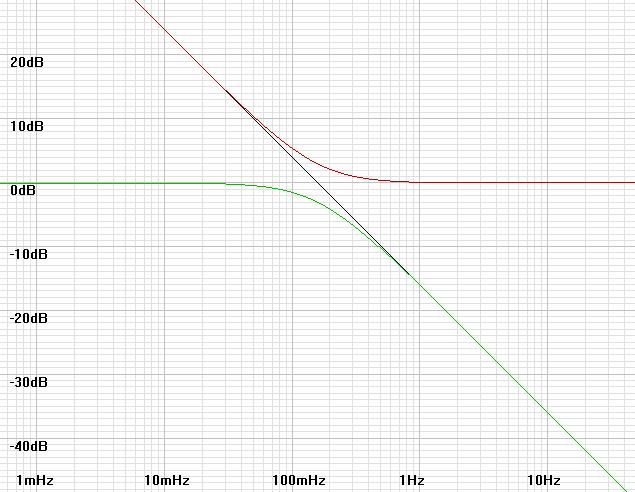Figure 2:  Non-inverting and inverting two stage first order servos.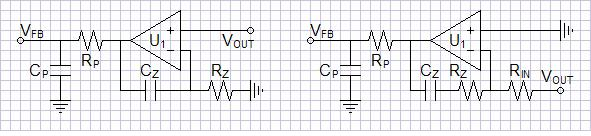A disadvantage of this design is that the output signal is present in full with boosted DC error signal at output of the integrator awaiting suppression by second stage.  Biasing of the servo must allow headroom for AC component.

#### Calculations

The final highpass pole will be at the frequency where |βservo| = |βglobal|.  β is feedback factor or gain of feedback loop.
To prove this assertion and give you useful servo equations, include both feedback factors in the closed-loop feedback gain equation:
 AV-CL = AV-OL 1+AV-OLβ = AV-OL 1+AV-OL(βglobal+βservo)
If the loop gain AV-OLβ >> 1 (usually met, except in low gain circuits) then the ideal gain equation becomes:
 AV-CL = AV-OL 1+AV-OL(βglobal+βservo) = AV-OL AV-OL(βglobal+βservo) = 1 (βglobal+βservo)
At the servo highpass pole, |βservo| = |βglobal| but their phases are 0º and -90º respectively.  The gain now becomes:
 AV-CL = 1 βglobal - jβservo = 1 βglobal(1 - j) , (j here is a vector number with a 90º degree direction)
1/(1 - j) in polar terms is 0.7071<45º the -3dB response of a first order highpass filter.
These equations ideally include the high-frequency effects that require compensation calculations, but here are used only as they affect servo operation.

The servo feedback factor is inversely proportional to frequency.  Where ω = 2πf = 1/T and ωpz is the frequency of the integrator zero and of the second stage pole:
 βservo = βservo0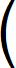ωpz ω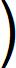= βservo0fpz fβservo0 represents servo gain at ωpz and is unity for the non-inverting circuit.  For the inverting circuit
 βservo0 = Rz Rin
You will have to multiply into βservo0 any other gain factors you may add.  A likely example is the addition of a voltage divider before the integrator in a power amp where the direct output voltage would overdrive the op amp.

Exact calculations depend on the particular setup.  Refer to the example below for details.

#### Example

As an example I will add a non-inverting servo of this type to the voltage feedback amplifier of Musical Feedback Amplifiers.  It will function in this circuit to automatically bias the amplifier as well as reduce offset.  Offset will not be as low as it would be if servo was not acting to bias the ciruit as well, but it should still be good.  As a desirable side effect, driving the circuit bias will put the op-amp in class A operation, reducing the urge to use separate power supplies.

The following circuit was unstable without compensation according to SPICE1 modeling.  With dominant pole compensation of pole frequency >= 20kHz, it showed stable results.  I tried poles approaching 100kHz with good results also.  Its asymmetrical design should ensure even and odd harmonics for those with that preference.

The design proceeds as follows:

• Make transistor choices.  (I chose LSK170A JFETs and 2N3906 PNPs for these analyses, other choices would work well too.)
• Decide power supply voltages.
• Choose RL (and R1 and R2 if used) for desired output impedance (in this case 1kΩ).
• Choose RBE to set bias through Q1.  Check Q1 datasheet for a proper value here.
• Choose CC for desired compensation pole frequency.  (Ordinarily this involves examining the loop gain (AV-OLβglobal) in great detail, here SPICE should show whether our pole chosen for musical reasons will result in oscillation.)
• Simulate circuit in SPICE with reasonable RS to negative power supply (RS = VSS/2ID will do) and adjust compensation if needed.
• Choose servo break frequency of 159.155mHz for even 1sec time constant.
• Choose op-amp.  Any will do that will operate on the chosen power supply.  High DC gain and low output offset are desired, other parameters are less critical, bandwidth can be low.  The LF411 is often chosen for this sort of application.
• Calculate RS to meet anticipated servo bias (i.e. terminated to -7.5V)
• Calculate R2S and R3S to drive desired servo bias from op-amp biased at output at 0V at reasonable current value within specs.
• Calculate feedback factors then calculate actual integrator pole and zero frequencies.
• From these calculate and choose remaining servo component values.
• Simulate and verify final circuit in SPICE.
Notes:
Output impedance in calculations is open-loop.  Closed loop output impedance will be reduced by the feedback factor (AOL/ACL).  Adding a small resistance (100Ω-1kΩ) on the output can give short circuit protection where an external cable is driven.

 Figure 3:  Simplest voltage-feedback op-amp with non-inverting feedback network and DC servo.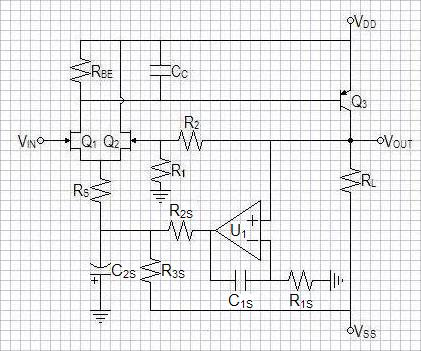Figure 3:  LSK170A SPICE model for reference .MODEL LSK170A NJF + BETA   = 0.0378643       VTO    = -0.4025156      LAMBDA = 4.783719E-3        + IS     = 3.55773E-14      + RD     = 10.6565         RS     = 6.8790487                    + CGD    = 3.99E-11        CGS    = 4.06518E-11        + PB     = 0.981382        FC     = 0.5                + KF     = 0               AF     = 1

Amplifier Design and Compensation Procedure

Choose LSK170A JFETs and 2N3906 PNPs for these analyses
Choose power supplies as +-15V.

ZOUT = RL || (R1 + R2) = 1kΩ.
Choose RL = 2kΩ, therefore R1 + R2 = 2kΩ.
For gain of 10, R1 = 200Ω, R2 = 1.8kΩ. (Ideal gain formula here is ACL = 1 + R2/R1)
iB-Q3 = -VSS/(hfe x ZOUT) = 15/(100 x 2kΩ) = 75µA.
LSK170A has min IDSS of 2.6mA, choose iD = 2mA and calculate RBE.
RBE = vbe/(iD - iB-Q3) = 700mV/(2mA - 75µA) = 363.6363636Ω.
Round up to nearest standard 5% value:  RBE = 360Ω.
rb-Q3 = VT/iB-Q3 = 25mV/75µA = 333.3333333Ω. (VT is the temperature dependent characteristic voltage of a PN junction, usually 25-26mV)
RPOLE = RBE || rb-Q3 = 360Ω || 333.3333333Ω = 173.0769231Ω
Calculate compensation capacitor for 20kHz dominant pole.
 CPOLE = 1 2πfPOLERPOLE = 1 2π x 20kHz x 173.0769231Ω = 45.97809467nF
Cb = Cibo + Cobo(A+1) = 10pF + 4.5pF(1kΩ/333.3333333Ω + 1) = 28pF (These from 2N3906 datasheet, multiplying Cobo by A+1 is the Miller effect)
CC = CPOLE - Cb, because CPOLE >> Cb, CC (approx.)= CPOLE = 45.97809467nF.
Choose standard value CC = 47nF.
ID  = 700mV/360Ω + 75µA = 2.01944mA
Calculate source output impedance rs
 rs = 1 gfs = 1 2 x sqrt(knID) = 1 2 x sqrt(37.8643mA/V2 x 2.01944mA) = 57.1794Ω

Servo Design Procedure
Choose break frequency of 159.155mHz for even 1sec time constant.
Choose LF411 operational amplifier.

Want first stage of servo at 0V DC bias to drive C2S of second stage to -7.5V
VGS = VT + sqrt(ID/kn) = -402.516mV + sqrt(2.01944mA/37.8643mA/V2) = -171.575mV
IRS = 2 x ID  = 4.03889mA
Calculate R terminated to -7.5V:
RS = (7.5V + |VGS|)/(iD x 2) = (7.5V + 171.575mV)/4.03889mA = 1.89943kΩ.
Round down to nearest 5%.  Choose RS = 1.8kΩ.
Calculate  R2S for 10mA op-amp output:
R2S = 7.5V/10mA = 750Ω.
R3S = 7.5V/(10mA + 4.03889mA) = 534.23Ω.
Round up to nearest 5%.  Choose R3S = 560Ω.
Now calculate to set servo break frequency.  Begin by calculating the feedback factors.
 βglobal = 200Ω 1.8kΩ + 200Ω = 0.1
Calculate additional drop in βservo from that at integrator zero frequency (now presuming resistive voltage divider drop in second stage)
 βservo-stage2 = 1.8kΩ || 560Ω 1.8kΩ || 560Ω + 750Ω = 427.119Ω 427.119Ω + 750Ω = 427.119Ω 427.119Ω + 750Ω = 362.851m(V/V)
Preliminary SPICE evaluation indicates I underestimated additional drop (pole frequency was much lower than expected).  Proceed now on the basis of inserting the servo feedback at the Q1 source rather than at the end of RS.  Calculate additional drop due to voltage divider between RS and rs presuming voltage dividers are close to independent due to scale of impedances:
 βatQ1Q2source = rs RS || rs = 57.1794Ω 1.8kΩ + 57.1794Ω = 30.7883m(V/V)
βservo0 =  βservo-stage2 x βatQ1Q2source = 362.851m(V/V) x 30.7883m(V/V) = 11.1716m(V/V)

Where ω = 2πf = 1/T:
 βglobal = 0.1 = βservo =  βservo0ωpz ω= βservo0 2πfhpTpz
Calculate servo pole/zero time constant from desired highpass break frequency:
 Tpz = βservo0 2πfhpβglobal = 11.1716m(V/V) 2π x 159.155mHz x 0.1 = 111.716msec
Rservo-pole-2ndst = RS || R2S || R3S = 1.8kΩ || 750Ω || 560Ω = 272.138Ω
C2S = Tpz/Rservo-pole-2ndst = 111.716msec/272.138Ω = 410.512µF
Round to nearest standard 5% value:  Choose C2S = 430µF.

Choose  R1S = 330kΩ and calculate C1S:
C1S =  Tpz/R1S = 111.716msec/330kΩ = 338.533nF
Round to nearest standard 5% value:  Choose C1S =  330nF.

#### SPICE Results

Output offset = -13.3143µV
Frequency response:  0.17378Hz - 660.7kHz  +0,-3dB (Deviation of servo pole from chosen 0.159155Hz is negligible)
SNR = 109.875dB
Integrator output bias:  -1.33143V (You would want to trim R2S or R3S (and perhaps C2S) if this value was too far from 0V)
Fourier analysis for vout @ 1kHz:
No. Harmonics: 10, THD: 0.0296942 %, Gridsize: 200, Interpolation Degree: 1

 Harmonic Frequency Magnitude Phase Norm. Mag Norm. Phase -------- --------- --------- ----- --------- ----------- 0 0 0 0 0 0 1 1000 1.01354 -90.074 1 0 2 2000 0.000300274 -164.01 0.000296263 -73.939 3 3000 2.02959e-005 -73.348 2.00248e-005 16.7261 4 4000 9.88967e-007 11.5644 9.75756e-007 101.639 5 5000 3.507e-007 -179.5 3.46015e-007 -89.429 6 6000 1.89024e-007 74.622 1.86499e-007 164.696 7 7000 5.40419e-007 -6.6935 5.332e-007 83.3809 8 8000 1.70615e-007 -125.83 1.68336e-007 -35.755 9 9000 6.05319e-007 160.262 5.97233e-007 250.337

SPICE Model for circuit of figure 31.

1Links to supporting SPICE models on this website: LSK170.txt, models1.txt

Document History
July 20, 2010  Created
July 21, 2010  Elaborated servo equations to better clarify integrator response.